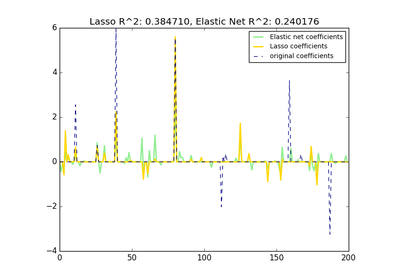# `sklearn.metrics`.r2_score¶

`sklearn.metrics.``r2_score`(y_true, y_pred, sample_weight=None, multioutput=None)[source]

R^2 (coefficient of determination) regression score function.

Best possible score is 1.0 and it can be negative (because the model can be arbitrarily worse). A constant model that always predicts the expected value of y, disregarding the input features, would get a R^2 score of 0.0.

Read more in the User Guide.

Parameters: y_true : array-like of shape = (n_samples) or (n_samples, n_outputs) Ground truth (correct) target values. y_pred : array-like of shape = (n_samples) or (n_samples, n_outputs) Estimated target values. sample_weight : array-like of shape = (n_samples), optional Sample weights. multioutput : string in [‘raw_values’, ‘uniform_average’, ‘variance_weighted’] or None or array-like of shape (n_outputs) Defines aggregating of multiple output scores. Array-like value defines weights used to average scores. Default value corresponds to ‘variance_weighted’, this behaviour is deprecated since version 0.17 and will be changed to ‘uniform_average’ starting from 0.19. ‘raw_values’ : Returns a full set of scores in case of multioutput input. ‘uniform_average’ : Scores of all outputs are averaged with uniform weight. ‘variance_weighted’ : Scores of all outputs are averaged, weighted by the variances of each individual output. z : float or ndarray of floats The R^2 score or ndarray of scores if ‘multioutput’ is ‘raw_values’.

Notes

This is not a symmetric function.

Unlike most other scores, R^2 score may be negative (it need not actually be the square of a quantity R).

References

Examples

```>>> from sklearn.metrics import r2_score
>>> y_true = [3, -0.5, 2, 7]
>>> y_pred = [2.5, 0.0, 2, 8]
>>> r2_score(y_true, y_pred)
0.948...
>>> y_true = [[0.5, 1], [-1, 1], [7, -6]]
>>> y_pred = [[0, 2], [-1, 2], [8, -5]]
>>> r2_score(y_true, y_pred, multioutput='variance_weighted')
0.938...
>>> y_true = [1,2,3]
>>> y_pred = [1,2,3]
>>> r2_score(y_true, y_pred)
1.0
>>> y_true = [1,2,3]
>>> y_pred = [2,2,2]
>>> r2_score(y_true, y_pred)
0.0
>>> y_true = [1,2,3]
>>> y_pred = [3,2,1]
>>> r2_score(y_true, y_pred)
-3.0
```

## Examples using `sklearn.metrics.r2_score`¶Lasso and Elastic Net for Sparse Signals